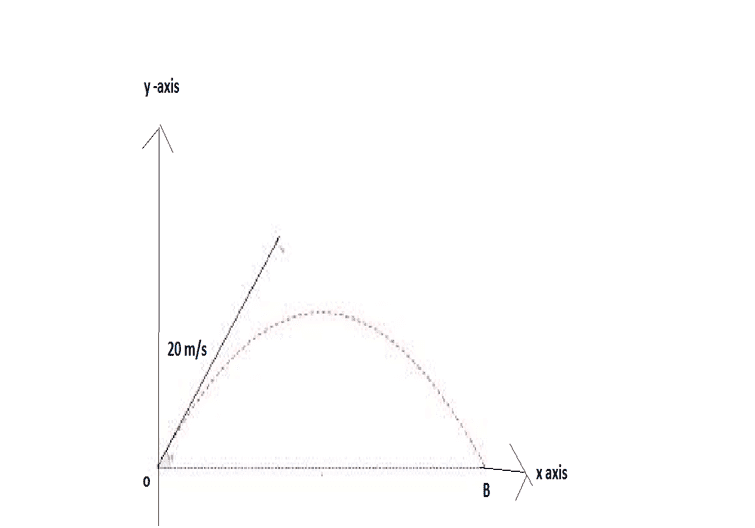# Typical projectile problem

## Homework Statement

There is a projectile which is launched at point "o" and land at "B".It was thrown with 20m/s with angle of 53 degree.Take g=10 m/s^2 sin theta=0.8 and cos theta=0.6
Find the time at which magnitude of x component of velocity of projectile is twice the magnitude of y component of velocity.## Homework Equations

v sub x =u sub x +a sub x t
u sub x=initial velocity in x-direction i.e u cos theta =16 m/s
v sub x =velocity in x direction at time t.
v sub y =velocity in y direction at time t
v sub y =u sin theta +(- 10 t)

## The Attempt at a Solution

v sub x=2 (u sin theta +(- 10 t)
but v sub x=u cos theta (as acceleration in x i.e horizontal direction is zero.
so,u cos theta =2 (u sin theta - 10 t)
putting given values give
t=1 sec.
But in book two answer are given
t=1 sec
and t=2.2 sec.
How I can get t=2.2 s ?

## Answers and Replies

Actually there would be two cases when horizontal velocity would be 2 times of vertical velocity as shown in your attempt.
Consider the case when projectile reaches highest point, calculate the time for it.
Then see the additional time where once again the horizontal vel. would be equal to 2 times the ver. Vel.

RUber
Homework Helper
You have to look at the return path, so try the negative.

SteamKing
Staff Emeritus
Science Advisor
Homework Helper

## Homework Statement

There is a projectile which is launched at point "o" and land at "B".It was thrown with 20m/s with angle of 53 degree.Take g=10 m/s^2 sin theta=0.8 and cos theta=0.6
Find the time at which magnitude of x component of velocity of projectile is twice the magnitude of y component of velocity.
View attachment 82093

## Homework Equations

v sub x =u sub x +a sub x t
u sub x=initial velocity in x-direction i.e u cos theta =16 m/s
v sub x =velocity in x direction at time t.
v sub y =velocity in y direction at time t
v sub y =u sin theta +(- 10 t)

## The Attempt at a Solution

v sub x=2 (u sin theta +(- 10 t)
but v sub x=u cos theta (as acceleration in x i.e horizontal direction is zero.
so,u cos theta =2 (u sin theta - 10 t)
putting given values give
t=1 sec.
But in book two answer are given
t=1 sec
and t=2.2 sec.
How I can get t=2.2 s ?

Find out how much time it takes for the ball to reach the top of the parabola and start falling back to earth.

In your equation:

vx = 2[u sin (θ) ± 10t],

you picked the negative sign and worked out t. What if you then picked the positive sign and solved this equation? What value of t would you get then?

What if you then picked the positive sign
Why?acceleration due to gravity is always pointing downward and I have chosen downward direction to be negative.

SteamKing
Staff Emeritus
Science Advisor
Homework Helper
Why?acceleration due to gravity is always pointing downward and I have chosen downward direction to be negative.
Well, then why did you write the equation with ± ?The first comment still applies. After the ball reaches its maximum height and starts falling again, there will be another point where the horizontal velocity is twice the verical velocity.•gracy
Well, then why did you write the equation with ± ?
No.I have not.I was just intended to write v=u+at as a is negative,it became v=u +(-a)t

The first comment still applies. After the ball reaches its maximum height and starts falling again, there will be another point where the horizontal velocity is twice the verical velocity.
I got your point.As there is symmetry ,there will be another point where the horizontal velocity is twice the vertical velocity.Thanks .